# (a) The band theory of solids can be used to qualitatively explain the difference in electrical...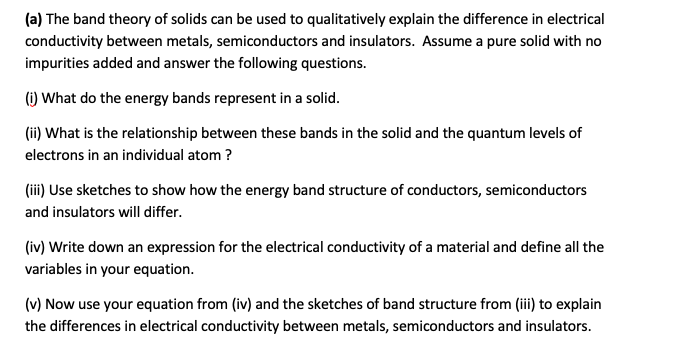(a) The band theory of solids can be used to qualitatively explain the difference in electrical conductivity between metals, semiconductors and insulators. Assume a pure solid with no impurities added and answer the following questions. (1) What do the energy bands represent in a solid. (ii) What is the relationship between these bands in the solid and the quantum levels of electrons in an individual atom? (ii) Use sketches to show how the energy band structure of conductors, semiconductors and insulators will differ. (iv) Write down an expression for the electrical conductivity of a material and define all the variables in your equation. (v) Now use your equation from (iv) and the sketches of band structure from (iii) to explain the differences in electrical conductivity between metals, semiconductors and insulators.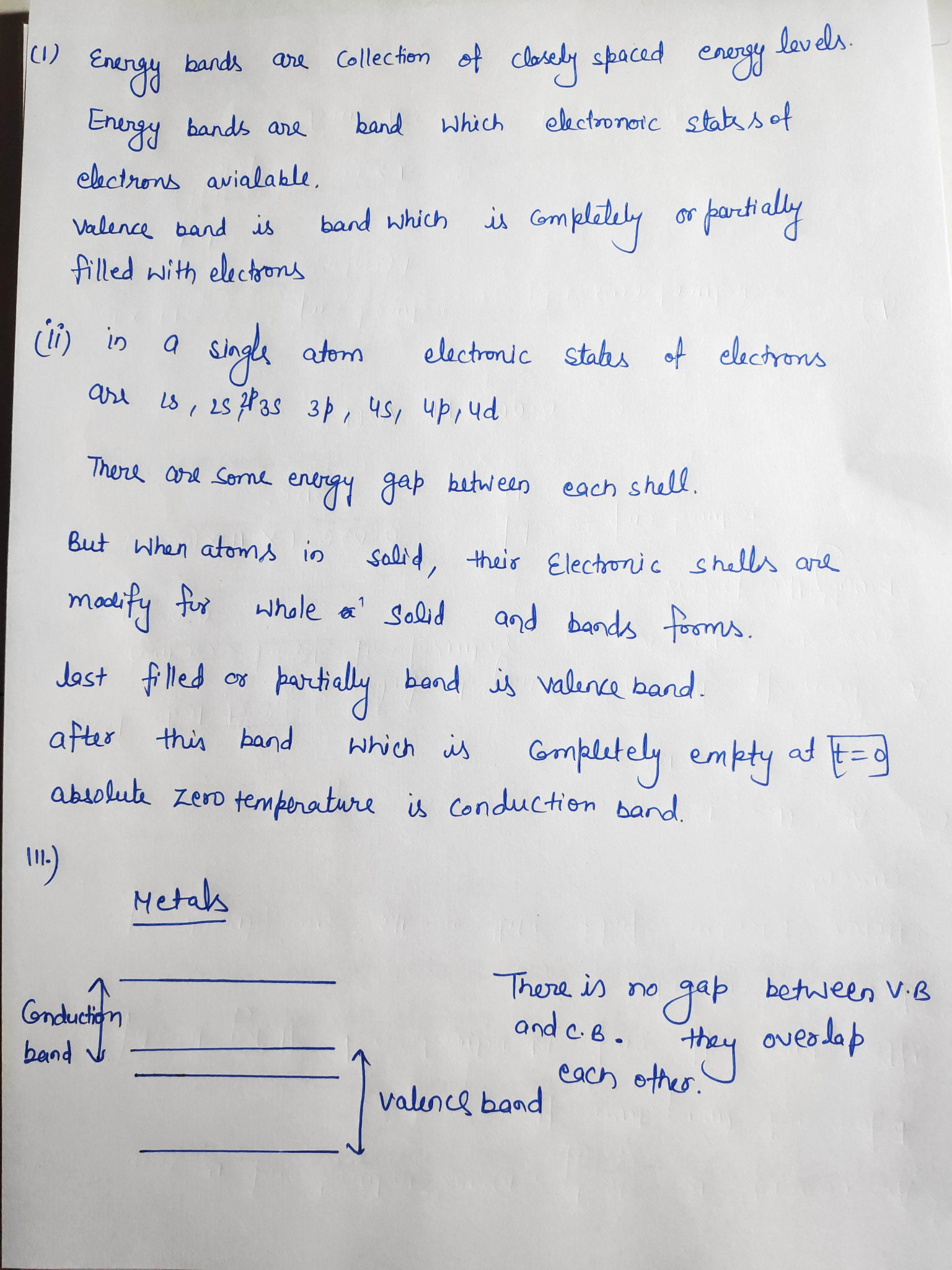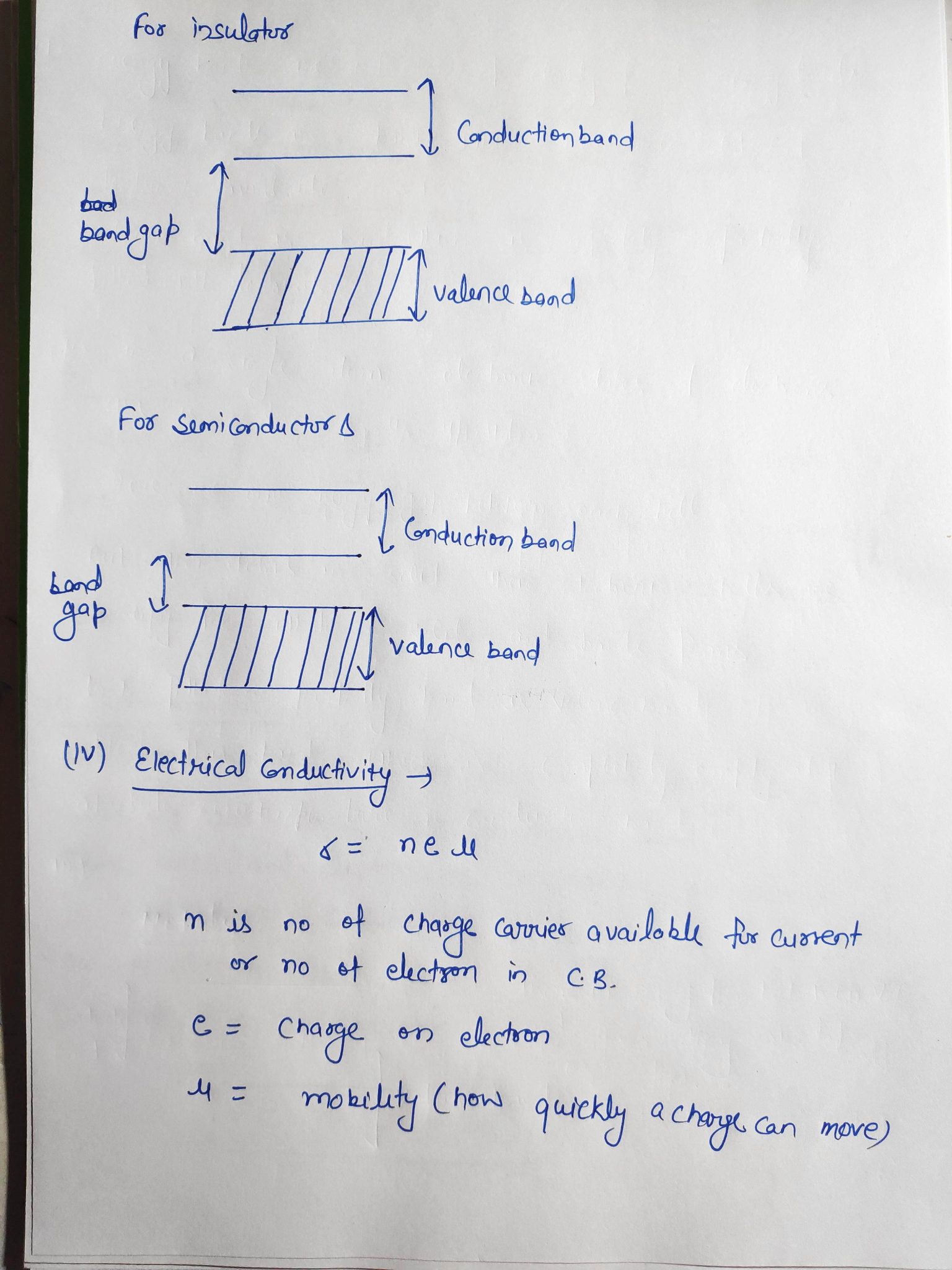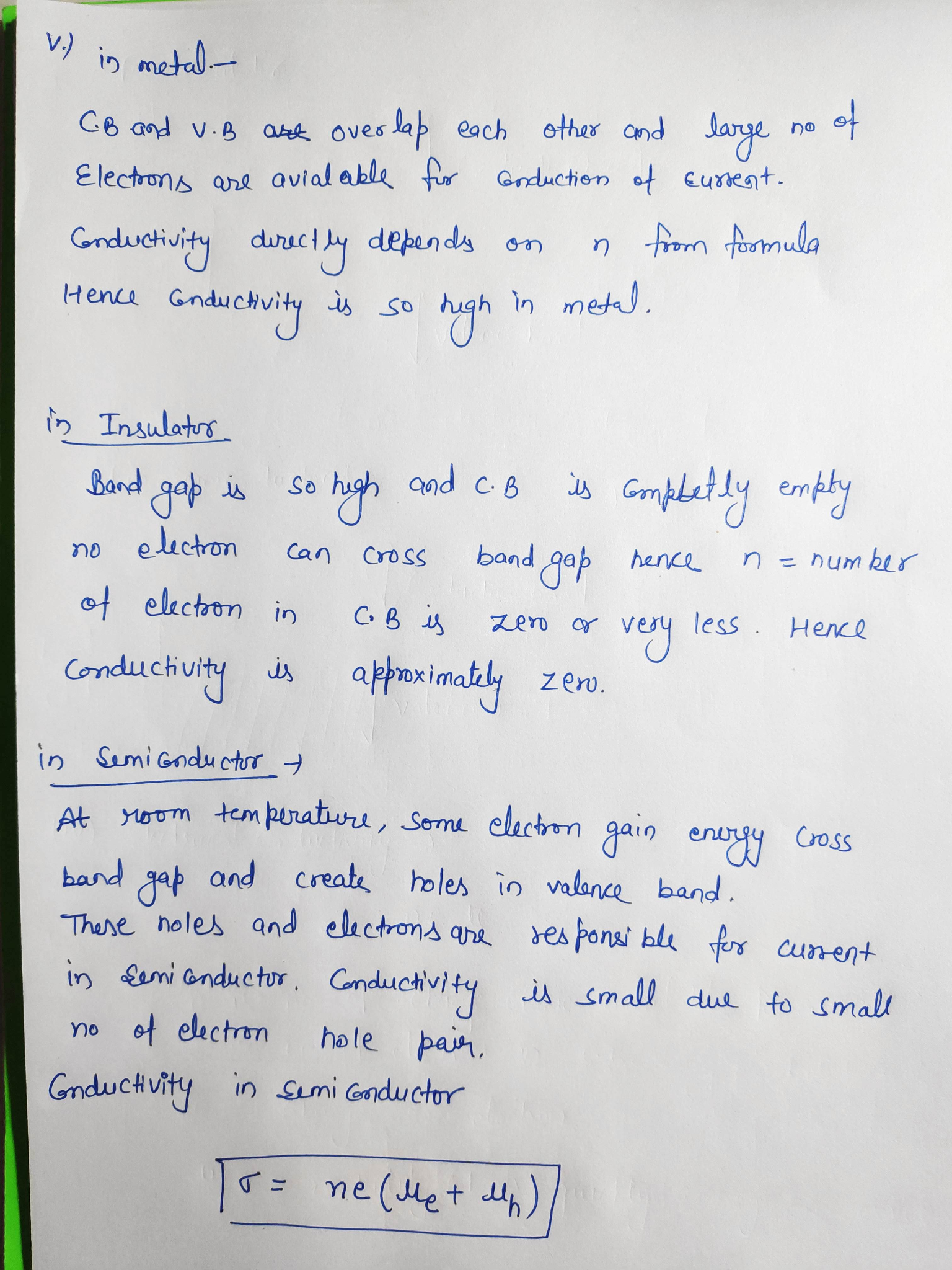##### Add Answer of: (a) The band theory of solids can be used to qualitatively explain the difference in electrical...
Similar Homework Help Questions
• ### (a) The band theory of solids can be used to qualitatively explain the difference in electrical...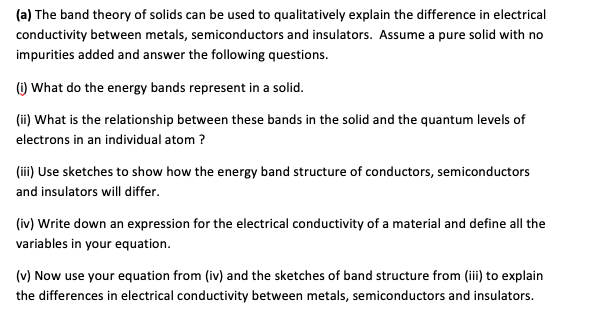(a) The band theory of solids can be used to qualitatively explain the difference in electrical conductivity between metals, semiconductors and insulators. Assume a pure solid with no impurities added and answer the following questions. ) What do the energy bands represent in a solid. (ii) What is the relationship between these bands in the solid and the quantum levels of electrons in an individual atom? (iii) Use sketches to show how the energy band structure of conductors, semiconductors and...

• ### (a) The band theory of solids can be used to qualitatively explain the difference in electrical...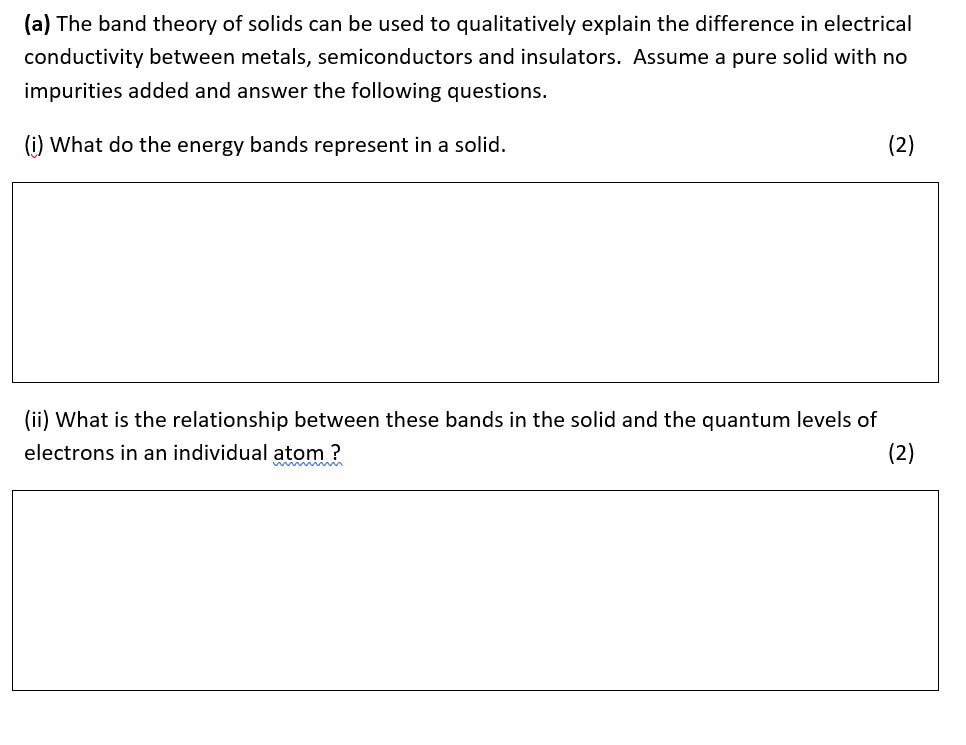(a) The band theory of solids can be used to qualitatively explain the difference in electrical conductivity between metals, semiconductors and insulators. Assume a pure solid with no impurities added and answer the following questions. (i) What do the energy bands represent in a solid. (2) (ii) What is the relationship between these bands in the solid and the quantum levels of electrons in an individual atom ? (2)

• ### (iv) Write down an expression for the electrical conductivity of a material and define all the...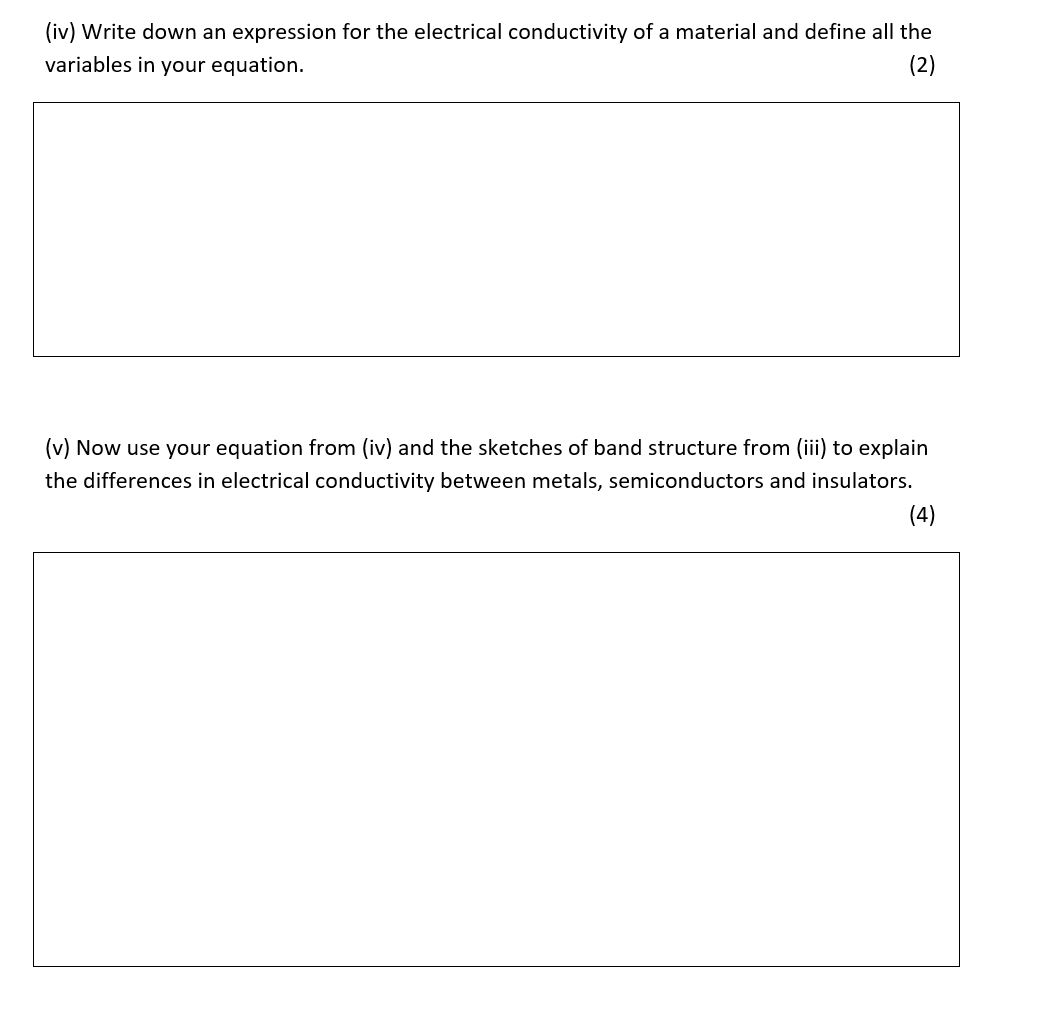(iv) Write down an expression for the electrical conductivity of a material and define all the variables in your equation. (2) (v) Now use your equation from (iv) and the sketches of band structure from (iii) to explain the differences in electrical conductivity between metals, semiconductors and insulators. (4)

• ### (iii) Use sketches to show how the energy band structure of conductors, semiconductors and insulators will...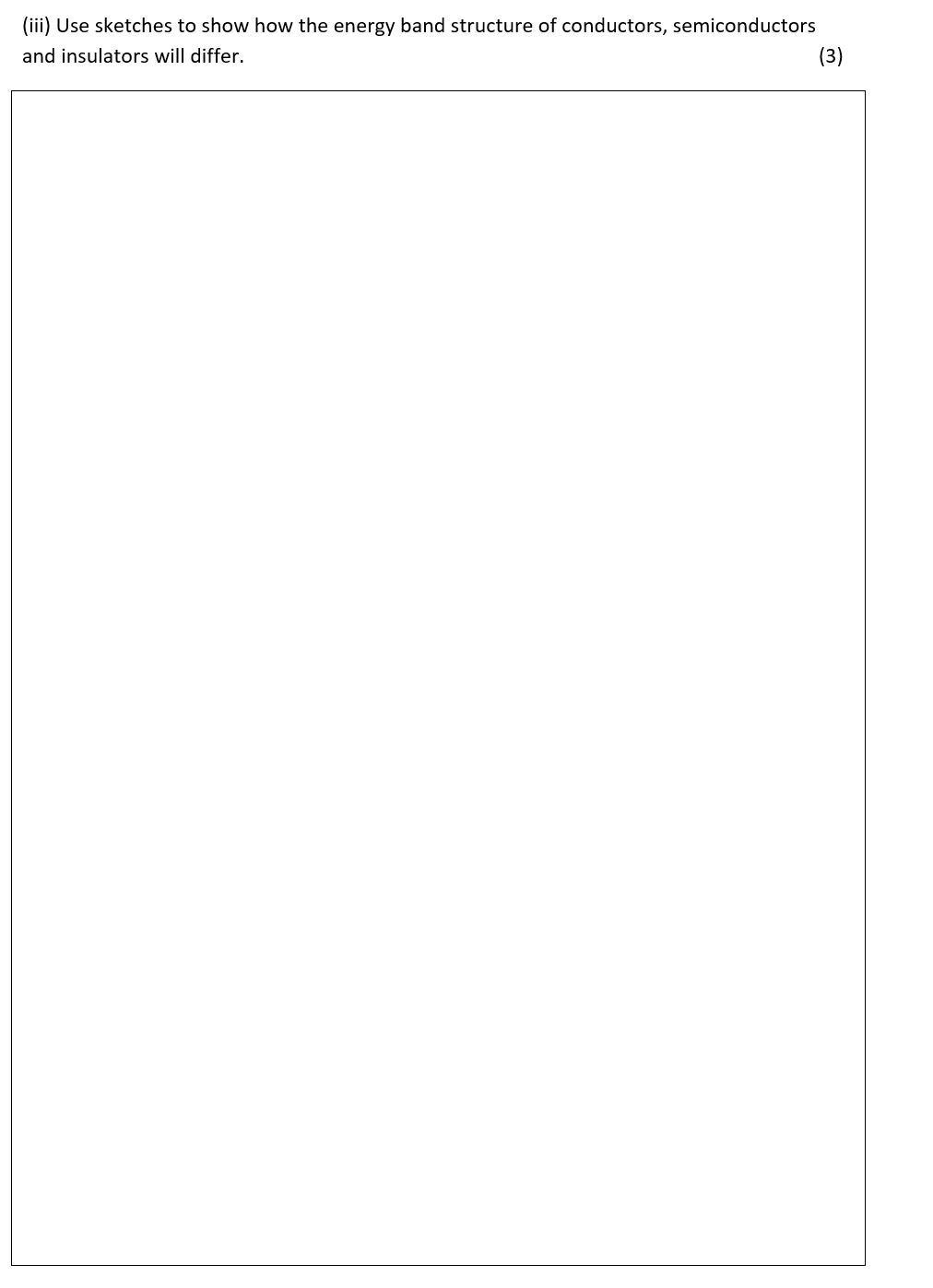(iii) Use sketches to show how the energy band structure of conductors, semiconductors and insulators will differ. (3)

• ### 5) Use the notion of energy bands to explain the following optical properties of solids: (a)...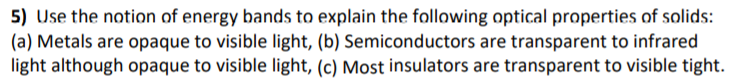5) Use the notion of energy bands to explain the following optical properties of solids: (a) Metals are opaque to visible light, (b) Semiconductors are transparent to infrared light although opaque to visible light, (c) Most insulators are transparent to visible tight.

• ### Q6. [20 Marks] (a) Write short notes on electrical insulators, conductors, and semiconductors (3mrk) (b) Write...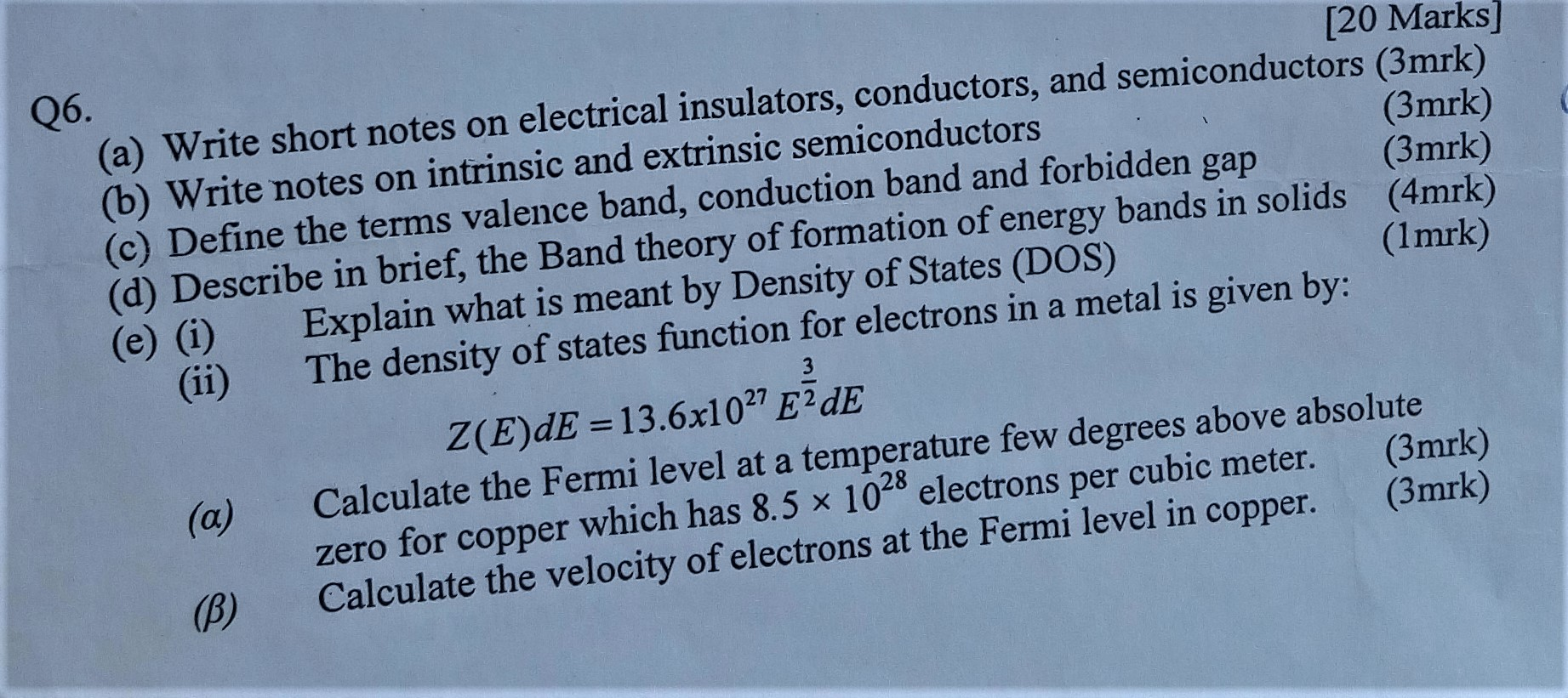Q6. [20 Marks] (a) Write short notes on electrical insulators, conductors, and semiconductors (3mrk) (b) Write notes on intrinsic and extrinsic semiconductors (3mrk) (c) Define the terms valence band, conduction band and forbidden gap (3mrk) (d) Describe in brief, the Band theory of formation of energy bands in solids (4mrk) (e) (i) Explain what is meant by Density of States (DOS) (1mrk) The density of states function for electrons in a metal is given by: Z(E)dE = 13.6x1027 E2 DE...

• ### 3.2 Periodic trends 1. (0620-5 2012-Paper 1/2-Q21) Which properties of the element titanium, Ti, can be...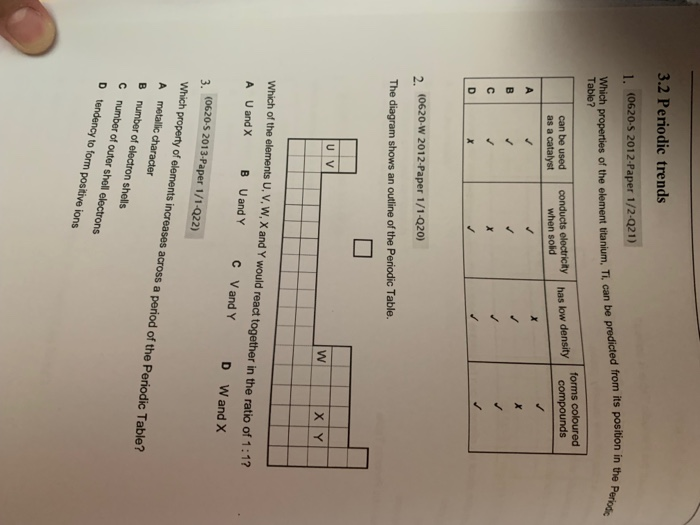3.2 Periodic trends 1. (0620-5 2012-Paper 1/2-Q21) Which properties of the element titanium, Ti, can be predicted from its position in the Periodic Table? forms coloured compounds conducts electricity when solid can be used has low density as a catalyst X A X X Xx 2. (0620-W 2012-Paper 1/1-Q20) The diagram shows an outline of the Periodic Table. U V W X Y Which of the elements U, V, W, X and Y would react together in the ratio of...

• ### I have a chemistry test that I need help with

I have a chemistry test that I need help with. My answers have stars by them Can someone please help me with the answers to this test??? Part 1 – Matter as Energy & Structure 1. When a pair of electrons is shared between two atoms, a covalent bond is said to have formed. Most often, the pair is formed a. by excess valence electrons b. as one atom contributes both electrons* c. as each atom contributes one electron d....